# How to write rho, uprho, varrho, and big rho symbol in LaTeX?

In LaTeX, the Greek letter rho, var rho, and its uppercase variant, big rho are commonly used in mathematical equations, physics, and other scientific disciplines.

LaTeX provides straightforward methods to insert these symbols into your documents.

In this tutorial, we will explore how to insert rho and big rho in LaTeX, along with examples of their usage.

## Insert rho symbol in latex documnet

To include the lowercase Greek letter “rho” in an equation or text, you can simply use the command \rho.

\documentclass[12pt]{article}
\usepackage[margin=1.5cm]{geometry}
\begin{document}
\section*{Use rho in density}
The density is represented by the symbol $\rho$ and is defined as mass divided by volume.
$\rho = \frac{m}{V}$
where:\\
- $\rho$ (rho) represents density of a substance,\\
- $m$ mass of substance,\\
- $V$ volume occupied by the substance.
\vspace{.5cm}
$\rho \left( \vec{r} \right) = \frac{dm}{dv}$
$m= \int_v \rho \left( \vec{r} \right) \, dv$
$\rho \left( \rho , v \right) = \sum_i \frac{v_i}{v}$

\section*{Use rho in resistivity }
The formula for resistivity is:
$\rho = \frac{R \times A}{L}$
where:\\
- $\rho$ (rho) represents the resistivity of the material,\\
- $R$ is the resistance of a uniform conductor made from that material,\\
- $A$ is the cross-sectional area of the conductor,\\
- $L$ is the length of the conductor.

\end{document}

Output :

## Use rho symbol in text

This symbol is not only used in mathematical expressions. Used in text. For this, the best method is to use inline math mode.

Additionally, Use of textrho command from textgreek package is shown.

\documentclass[12pt]{article}
\usepackage{textgreek}
\begin{document}
\section*{Use inline math mode like ($\rho$).}
Rho ($\rho$) plays a significant role in the field of fluid dynamics, where it is used to calculate flow rates, pressure drops, and other properties of fluids in pipes and channels.
\section*{Use textrho(\textrho) cmd from textgreek pkg}
In mathematics, \textrho \, can represent a complex number in polar form, where \textrho \, is the magnitude and the angle $\theta$ represents the argument or phase.
\end{document}

Output :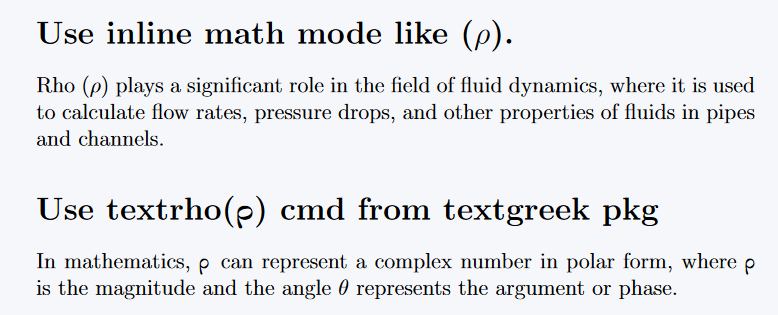## Use of uprho symbol

Many of you are not familiar with the uprho symbol. If you look at normal rho, you will see that the body is slightly curved. For this symbol, the body will be straight, much like the English letter p.

There is no default command for this, the external package needs to be inserted into preamble.

\documentclass[12pt]{article}
\usepackage{txfonts,upgreek, bm}
\begin{document}
$\rho \quad \rhoup \quad \uprho$
$x= \rhoup \cos{\phi}, \; y= \rhoup \sin{\phi}$
$\rhoup = \uprho^2, \; \rhoup(t)=G(t)\uprho(0)G(t)$
$\boldsymbol{r} = \boldsymbol{\rhoup\hat{\rhoup}} + \boldsymbol{z\hat{z}} \quad \uprho_q(\mathbf{r}) = q|\psi(\mathbf{r})|^2$
$\rhoup_q(\mathbf{r}) = \sum_{i=1}^N q_i\delta(\mathbf{r}-\mathbf{r}_i)$
\end{document}

Output :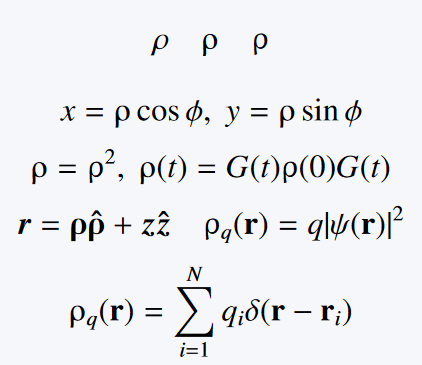## Use of var rho symbol

Var rho is rarely used, but good to know latex syntax. In this case, default command is present.

\documentclass[12pt]{article}
\usepackage{bm}
\begin{document}
$\rho \; and \; \varrho$
$\varrho^{+}, \varrho^{-}, \varrho^{0}$
$\bm{\varrho} = \sum_{i=1}^n \varrho_i \hat{\bm{\varrho}}$
\end{document}

Output :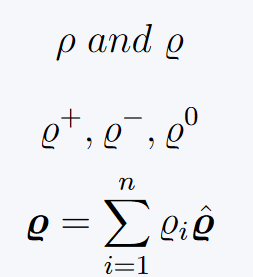## Use symbol in bold format

Do not convert Greek symbols to bold by the \mathbf{arg} command. There are other commands for this. Below will be a slight difference in the structure of the output by the three commands.

\documentclass[12pt]{article}
\usepackage{physics,bm,amsmath}
\begin{document}
$\mathbf{\rho}, \bm{\rho}, \pmb{\rho}, \boldsymbol{\rho}$
$\nabla f =\frac{\partial f}{\partial d} \boldsymbol{\hat{\rho}} + \frac{1}{\rho} \frac{\partial f}{\partial \psi}\boldsymbol{\hat{\psi}} + \frac{\partial f}{\partial z}\boldsymbol{\hat{z}}$
$\bm{v} = \dot{\rho}\boldsymbol{\hat{\rho}} + \rho\dot{\varphi}\boldsymbol{\hat{\varphi}} + \dot{z}\boldsymbol{\hat{z}}$
\end{document}

Output :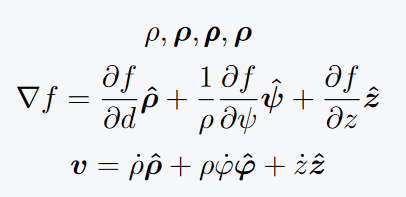Now we will see the use of superscript and subscript. Although the syntax is simple, large mathematical expressions can seem complicated. But, it is represented simply in the example below.

\rho^{arg} % Add only superscript
\rho^{arg}_{arg} % Add both
\documentclass{article}
\begin{document}
$\rho_x ,\, \rho_y , \, \rho_z ,\, \rho_{ij}$
$E_i = \rho_{ij} J_i$
$\rho^+,\, \rho^0,\, \rho^-,\,\mathrm{tr}\left(\rho^2\right) = 1$
$\rho_i' = \frac{P_i' \rho P }{\mathrm{tr}[P \rho P_i]'}$
\end{document}

Output :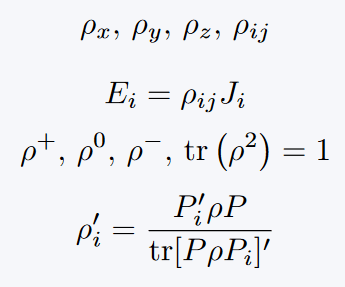## Conclusion

In conclusion, LaTeX provides simple methods to insert rho and big rho symbols into your mathematical equations and text.

Whether you need the lowercase “rho” symbol or the uppercase “big rho” symbol, LaTeX’s commands make it easy to include these Greek letters in your documents.#### Md Jidan Mondal

LaTeX expert with over 10 years of experience in document preparation and typesetting. Specializes in creating professional documents, reports, and presentations using LaTeX.